###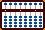Find the GCD and LCM of two numbers

** Before starting these techniques it's important to understand basic Japanese soroban operations **

GCD: The greatest common divisor (gcd) is also known as the greatest common factor (gcf) and the highest common factor (hcf). The GCD of two numbers is the largest factor that can divide into both numbers. (Note: if the GCD of two numbers equals 1, they are said to be coprime.)

The GCD for 12 and 18
Prime factors for 12 are: 2 x 2 x 3
Prime factors for 18 are: 2 x 3 x 3
Prime factors shared by 12 & 18 are 2 and 3
The greatest common divisor for 12 and 18 is 6

LCM: The least common multiple is sometimes also known as the lowest common multiple (lcm). The LCM of two numbers is the smallest number (not zero) that is a multiple of both numbers.

The LCM for 12 and 18
Multiples of 12 are: 0, 12, 24, 36, 48, 60, 72...
Multiples of 18 are: 0, 18, 36, 54, 72, 90, 108...
Both 12 and 18 share the multiples 36,  72  & ...
But the lowest common multiple shared by both is 36

Fortunately there is a very simple and efficient way to solve both GCD and LCM for two numbers. The following soroban techniques were shown to me by Enriello and by Edvaldo Siqueira both of whom are members of the Soroban / Abacus newsgroup. To find the GCD and LCM of two numbers (a, b) the techniques work like this:

1. Set the two numbers (a, b) onto the left side of soroban.
2. Set the first number (a) one more time to the right.
3. Working on the right, multiply a*b and add the product to the frame.
4. Using Euclid's algorithm*, find the GCD of a, b on the left.
5. Use the product calculated in step 3 to find the LCM. LCM = a*b / GCD.

*Euclid's algorithm is easily performed on an abacus. Start by subtracting the smaller number from the larger as many times as possible. In the event of a remainder, turn the process around and subtract the new smaller number from the larger. This process continues back and forth until only one number remains. The remaining number is the GCD. (Note: If at any point the numbers are radically different in size, rather than subtraction, division may be a better option.)

Example 1: Find the GCD and LCM for 12 & 18

Step 1: Set 12 on rods AB and 18 on rods DE. Set another 12 on rods JK. (Fig.1)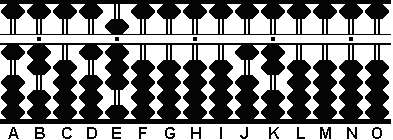Fig. 1 ```Step 1 A B C D E F G H I J K L M N O . . . . .   1 2 0 1 8 0 0 0 0 1 2 0 0 0 0```

Step 2: Multiply 12 on rods JK by 18 on DE. Add the product 216 to rods LMN. (Fig.2)Fig. 2 `Step 2` ```A B C D E F G H I J K L M N O . . . . .   1 2 0 1 8 0 0 0 0 0 0 2 1 6 0```

Find the GCD of 12 and 18 using Euclid's algorithm

Step 3: Subtract 12 from 18 on rods DE. The result is 6. (Fig.3)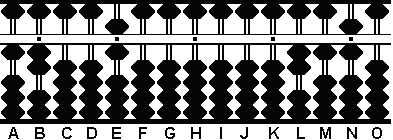Fig. 3 `Step 3` ```A B C D E F G H I J K L M N O . . . . .   1 2 0 0 6 0 0 0 0 0 0 2 1 6 0```

Step 4 & the GCD: Turn the process around and subtract 6 from 12 on rods AB. This can be done twice resulting in zero. Since there can be no more subtractions, we know the greatest common divisor for the problem is 6 on rod E. (Fig.4)Fig. 4 `Step 4` ```A B C D E F G H I J K L M N O . . . . .   0 0 0 0 6 0 0 0 0 0 0 2 1 6 0```

Now find the LCM by dividing 216 on rods LMN by 6 on rod E.

Step 5 & the LCM: Divide 6 on rod E into 216 on rods LMN. With this last step we know the least common multiple for 12 & 18 is the quotient answer 36 on rods KL. (Fig.5)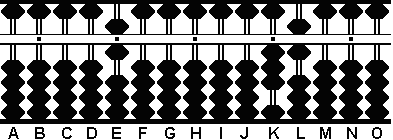Fig. 5 `Step 5` ```A B C D E F G H I J K L M N O . . . . .   0 0 0 0 6 0 0 0 0 0 3 6 0 0 0 .<- * * Kojima teaches; move the decimal 1 place + 1 to the left for every whole number in a divisor.```

Answers: The GCD of 12 and 18 = 6
:  The LCM of 12 and 18 = 36

Example 2: Find the GCD and LCM for 39 & 91

Step 1: Set 39 on rods AB and 91 on rods DE. Set another 39 on rods JK. (Fig.6)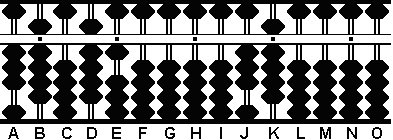Fig. 6 ```Step 1 A B C D E F G H I J K L M N O . . . . .   3 9 0 9 1 0 0 0 0 3 9 0 0 0 0```

Step 2: Multiply 39 on rods JK by 91 on DE. Add the product 3549 to rods KLMN. (Fig.7)Fig. 7 `Step 2` ```A B C D E F G H I J K L M N O . . . . .   3 9 0 9 1 0 0 0 0 0 3 5 4 9 0```

Find the GCD of 39 and 91 using Euclid's algorithm

Step 3: Subtract 39 rom 91 on rods DE. This can be done twice resulting in 13. (Fig.8)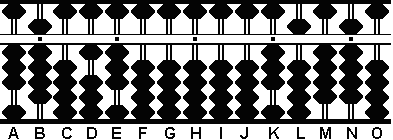Fig. 8 `Step 3` ```A B C D E F G H I J K L M N O . . . . .   3 9 0 1 3 0 0 0 0 0 3 5 4 9 0```

Step 4 & the GCD: Turn the process around and subtract 13 from 39 on rods AB. This can be done three times resulting in zero. Since there can be no more subtractions, we know the greatest common divisor for the problem is 13 on rods DE. (Fig.4)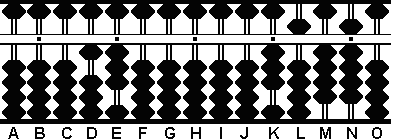Fig. 9 `Step 4` ```A B C D E F G H I J K L M N O . . . . .   0 0 0 1 3 0 0 0 0 0 3 5 4 9 0```

Now find the LCM by dividing 3549 on rods KLMN by 13 on rod DE.

Step 5 & the LCM: Divide 13 on rods DE into 3549 on rods KLMN. With this last step we know the least common multiple for 39 & 91 is the quotient answer 273 on rods IJK. (Fig.5)Fig. 10 `Step 5` ```A B C D E F G H I J K L M N O . . . . .   0 0 0 1 3 0 0 0 2 7 3 0 0 0 0 .<- - * * Kojima teaches; move the decimal 1 place + 1 to the left for every whole number in a divisor.```

Answers: The GCD of 39 and 91 = 13   :  The LCM of 39 and 91 = 273

##### LCM @ math is fun & GCD @ math is fun

 REFERENCES: Wikipedia Euclidean algorithm Greatest Common Divisor Least Common Multiple SOROBAN TECHNIQUES: Kojima, Takashi. The Japanese Abacus: Its Use and Theory Tokyo: Charles E. Tuttle, 1954 Tani, Yukio The Magic Calculator: the way of the abacus Tokyo: Japan Publications Trading Co, 1964 THANKS TO: Edvaldo Siquiera Enriello Gary Flom Euclid of Alexandria (ca. 325 BC-265 BC) Members of the Soroban / Abacus Newsgroup https://groups.io/g/sorobanabacus/ Print Page (.pdf format,161kb)

© April, 2006
Totton Heffelfinger  Toronto Ontario  Canada

Email
totton[at]idirect[dot]com